Courses

# Test: Physics- 7

## 45 Questions MCQ Test NEET Mock Test Series | Test: Physics- 7

Description
This mock test of Test: Physics- 7 for NEET helps you for every NEET entrance exam. This contains 45 Multiple Choice Questions for NEET Test: Physics- 7 (mcq) to study with solutions a complete question bank. The solved questions answers in this Test: Physics- 7 quiz give you a good mix of easy questions and tough questions. NEET students definitely take this Test: Physics- 7 exercise for a better result in the exam. You can find other Test: Physics- 7 extra questions, long questions & short questions for NEET on EduRev as well by searching above.
QUESTION: 1

### The sun subtends an angle of half a degree at the pole of a concave mirror which has a radius of curvature of 15 metres. Then the size (disameter) of the image of the sun formed by the concave mirror is

Solution:

θ = (1/2)o

= [π/2]/180

The image of sun is formed at focus.
θ = d/f

⇒ d = fθ

= 15/2 × π/360

= 0.0654m
=6.54cm

QUESTION: 2

### A ray of light strikes a plane mirror PQ at an angle of incidence of 30∘, is reflected from the plane mirror QR placed at right angles to the first mirror. The angle of reflection at the second mirror is: Draw a ray diagram to illustrate your answer.

Solution:

Ray AB strikes the mirror PQ at B and gets reflected along BC according to the laws of reflection. The ray BC incident on mirror QR makes an angle 300 with the mirror. So, the angle of incidence on this mirror is 900 −300 = 600 . Hence, the angle of reflection is also 600

QUESTION: 3

### A ray of ligth makes an angles of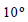with the horizontal and strikes a plane mirror which is inclined at an angle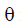to the horizontal.The anglfor which the reflected ray becomes vertical, is

Solution: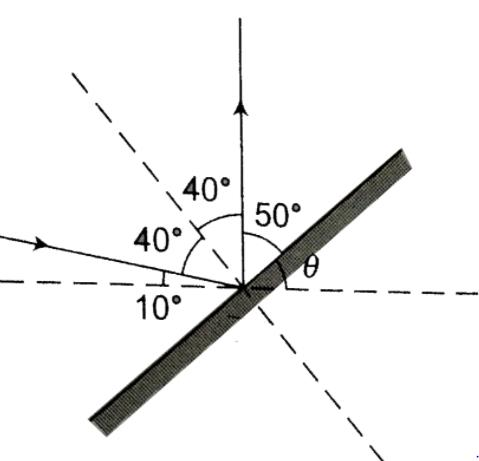50o + θ = 90o
θ=40o

QUESTION: 4
A motor car is fitted with a convex driving mirror of focal length 20 cm. A second motor car 2 m broad and 1.6 m high is 6 m away from the first car. Then the position of the second car as seen in the mirror of the first car is
Solution:
QUESTION: 5
Figure shows two rays A and B being reflected by a mirror and going as A’ and B’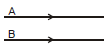the mirror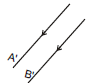Solution: A and B are parallel rays and after reflection A' and B' also parallel, that's happen when rays reflected by plane mirror. in other case after reflection rays will not be parallel.
QUESTION: 6

If the critical angle for total internal reflection from a medium to vacuum is 30°
the velocity of light in the medium is

Solution:
QUESTION: 7
Two immiscible liquids are in a cylindrical vessel of depths of 8.4 cm and 7.8 cm, their refractive indices being 1.2 and 1.3 respectively. An object O at the bottom isseen from vertically above the liquids. The upward displacement in cm
in its apparant position is
Solution:
QUESTION: 8

The minimum deviation for a ray of light through a prism is 44° and when this happens the angle of incidence is  50° The refracting angle of the prism is

Solution:
QUESTION: 9
A cube of side a made of a material of refractive index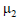is immersed in a liquid of refractive index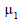A ray is incident on face AB at an angleas shown. Total internal reflection just takes place at point P on face BC. Then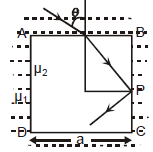Solution:
QUESTION: 10
X is a small angled prism of angle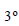made of a material of refractive index 1.5. A ray of light is made incident as shown in figure. M is a plane mirror.The angle of deviation for the ray reflected from the mirror. M with respect to incident ray is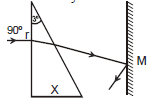Solution:
QUESTION: 11
The face AC of a prism ABC of refracting angle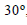is silvered. A ray is incident on face AB at an angle of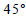as shown in figure. The refracted ray undergoes reflection on face AC and retraces its path.The refractive index of the prisms is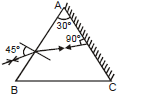Solution:
QUESTION: 12
If half the body of a lens is covered with black paper the image produced by the lens will
Solution:
QUESTION: 13
When a lens is inserted between an object and a screen which are at a fixed distance apart, the size of the image is either 6 cm, or 2/3 cm. The size of the object in cm is
Solution:
QUESTION: 14
What is the focal length of a double convex lens for which the radius of curvature of each surface is 40cm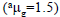?
Solution:
QUESTION: 15
An object 20 cm high produces using a lens a real image of 80cm.When an object is shifted by 5 cm a real image 40 cm high is produced.The power of the lens is
Solution:
QUESTION: 16

When the object is at a distance x1 and x2, a real and a virtual image of the same size are formed respectively. The focal length of the convex lens is

Solution:
QUESTION: 17
The graph shows the variation of magnification M produced by a convex thin lens with image distance V. What was the focal length of the lens used ?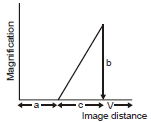Solution:
QUESTION: 18
A thin symmetric convex lens of refractive index of the material 1.5 and radius of curvature 0.5 metre is immersed in water of refractive index 4/3. Its focal
length will be
Solution:
QUESTION: 19
An isotropic source of 2 candela produces a light flux equal to
Solution:
QUESTION: 20
A lamp is hanging at a height 40 cm from the centre of a table. If its height is increased by 10 cm, the illuminance on the table will decrease by
Solution:
QUESTION: 21
The intensity of light at a distance r from the axis of a long cylindrical source is proportional to
Solution:
QUESTION: 22
Chromatic aberrations in the formation of images by a lens arise because
Solution:
QUESTION: 23
The focal length of a lens of flint glass of dispersive power 0.45 which will render achromatic a converging lens of crown glass of focal length 75cm and dispersive power 0.21 is
Solution:
QUESTION: 24
The magnifying power of telescope can be increased by
Solution:
QUESTION: 25
A movie projector projects an image of length 3.5 m of a 35 mm. movie film on a screen. Neglecting absorption of light by the film and the air, the ratio of the illumination on the screen is
Solution:
QUESTION: 26
The ƒ number of a camera lens is 4.5. Which of the following statements is correct ?
Solution:
QUESTION: 27
A n a s t r o n o mi c a l t e l e s c o p e h a s a n a n gu l a r magnification of magnitude 5 for distant objects. The separation between the objective and the eye piece is 36 cm and the final image is formed at infinity. The focal length of the objective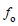and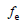of the eye piece are
Solution:
QUESTION: 28
The focal length of a simple convex lens used as a magnifier is 10 cm. For the image to be formed at a distance of distinct vision (D = 25 cm), the object
must be plced away from the lens at a distance of
Solution:
QUESTION: 29

A person can see objects only at distance greater than 40 cm. He is advised to use lens of power

Solution:
QUESTION: 30
The resolution limit of eye is 1 minute. At a distance of x km from the eye, two persons stands with a lateral separation of 3 metres. For the two persons
to be just resolved by the naked eye, x should be
Solution:
QUESTION: 31
In Young’s double slit experiment, we get 62 fringes in the field of view if we use sodium lamp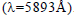How many fringes we will get in the same field of view if we use green lightSolution:
QUESTION: 32
Two polaroids are parallel to one another and making an angle ofwith the direction of a plane polarised beam A third polaroid, with the axis of transmission
at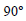with that of the first is placed. The fraction of incident light transmitted through all the three polaroids (assuming no losses) is
Solution:
QUESTION: 33
Polarisation of light proves the
Solution:
QUESTION: 34
Laser light is considered to be coherent because it consists of
Solution:
QUESTION: 35
The first diffraction minima due to a single slit diffraction is at=for a light of wavelength 5000Å. The width of the slit is
Solution:
QUESTION: 36
Polaroid glass is used in sunglasses because
Solution:
QUESTION: 37
The velocity of light is maximum in
Solution:
QUESTION: 38
Pick out the longest wavelength from the following types of radiations
Solution:
QUESTION: 39
A ray of light of intensity I is incident on a parallel glass-slab at point A as shown in figure. It undergoes partial reflection and refraction. At each reflection
25% of incident energy is reflected. The rays AB and A’B’ undergo interference. The ratio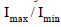is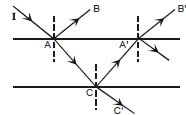Solution:
QUESTION: 40
In the Young’s double slit experiment a mica slip of thickness t and refractive index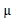is introduce in the ray from the first source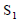By how much distance
the fringe pattern will be displaced
Solution:
QUESTION: 41
Which one of the following statement is wrong ?
Solution:
QUESTION: 42
Finger prints on paper are identified by sprinkling flourescent powder on it and observing it under
Solution:
QUESTION: 43
For a long cylindrical source of light, the illuminence E on a screen varies with distance r as
Solution:
QUESTION: 44
A convex lens of focal length 40 cm is held coaxially and 12 cm above a concave mirror of focal length 18 cm. A luminous point object placed d cm above
the lens on its axis gives rise to an image coincident with itself. Tehn d is equal to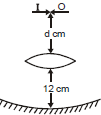Solution:
QUESTION: 45
The refracting angle of a prism is A and refractive index of material of a prism is cot A/2. The angle of minimum deviation is
Solution:

Track your progress, build streaks, highlight & save important lessons and more!

### Similar Content### Related tests